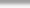## RANDOM numbers in ksh or ksh93

Here's are an example of a random number generator in KSH or it's newer counterpart KSH93.  For this post, we'll use the latest KSH from AT&T Labs:

• Generate a random set of integers between 0 – 256 and use them to create a random set of IP type entries:

#!/bin/ksh93u+

for (( KEY=0; KEY < 1000000000; KEY++ )); do
print \$(( RANDOM / 128 ))"."\$(( RANDOM / 128 ))"."\$(( RANDO^C/ 128 ))"."\$(( RANDOM / 128 ));
done

The purpose of the above is to obtain a random set of entries (IP like) for testing purposes in other logic including testing regular expressions.  RANDOM, in this case generates a number between 0 and 32768 and when divided by 128, will print out the intiger part of the equation.  To assign a random number a statement similar to MVAR=\$RANDOM; can also be used.

Cheers,
TK